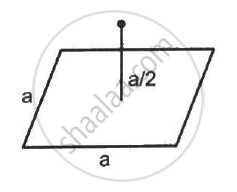Department of Pre-University Education, KarnatakaPUC Karnataka Science Class 12
Advertisement Remove all ads

# A Charge Q is Placed at a Distance A/2 Above the Centre of a Horizontal, Square Surface of Edge a as Shown in the Following Figure . Find the Flux of the Electric Field Through the Square Surface. - Physics

Answer in Brief

A charge Q is placed at a distance a/2 above the centre of a horizontal, square surface of edge a as shown in the following figure . Find the flux of the electric field through the square surface.Advertisement Remove all ads

#### Solution

Given:-
Edge length of the square surface = a
Distance of the charge Q from the square surface = a/2
Area of the plane = a2
Assume that the given surface is one of the faces of the imaginary cube.
Then, the charge is found to be at the centre of the cube.
A charge is placed at a distance of about "a"/2 from the centre of the surface.
The electric field due to this charge is passing through the six surfaces of the cube.
Hence flux through each surface,

phi = "Q"/∈_0 xx 1/6 = "Q"/(6∈_0)

Thus, the flux through the given surface is "Q"/(6∈_0).

Concept: Electric Charges
Is there an error in this question or solution?
Advertisement Remove all ads

#### APPEARS IN

HC Verma Class 11, Class 12 Concepts of Physics Vol. 2
Chapter 8 Gauss’s Law
Q 6 | Page 141
Advertisement Remove all ads

#### Video TutorialsVIEW ALL 

Advertisement Remove all ads
Share
Notifications

View all notifications

Forgot password?